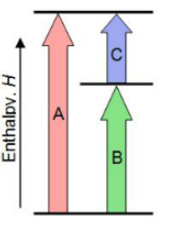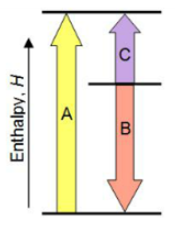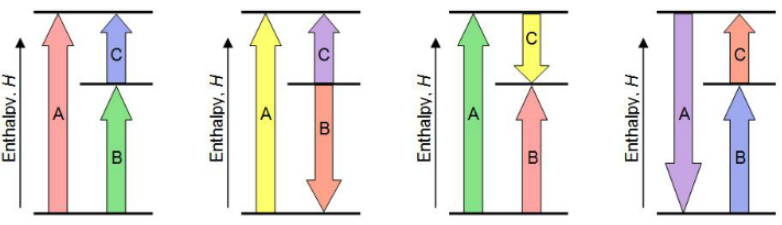# Problem: Match each chemical reaction with the correct diagram according to Hess' LawΔHC = -ΔHA + ΔHB ΔHB  = ΔHA  + ΔHCΔHA = ΔHB - ΔHCΔHB  = -ΔHA - ΔHC ΔHA = -ΔHB + ΔHCΔHC = ΔHA  + ΔHB ΔHB  = ΔHA - ΔHC ΔHC = -ΔHA - ΔHB

###### FREE Expert Solution

Assuming:

Upward → positive (+) value of ΔH

Downward → negative (-) value of ΔHΔHA = ΔHB + ΔHC

ΔHA = ΔHB + ΔHC
-ΔHC           - ΔHC

ΔH = ΔHA - ΔHC###### Problem Details

Match each chemical reaction with the correct diagram according to Hess' LawΔHC = -ΔH+ ΔH

ΔH = ΔHA  + ΔHC

ΔH= ΔH- ΔHC

ΔH = -ΔH- ΔHC

ΔH= -ΔH+ ΔHC

ΔH= ΔHA  + ΔH

ΔH = ΔH- ΔHC

ΔHC = -ΔH- ΔH